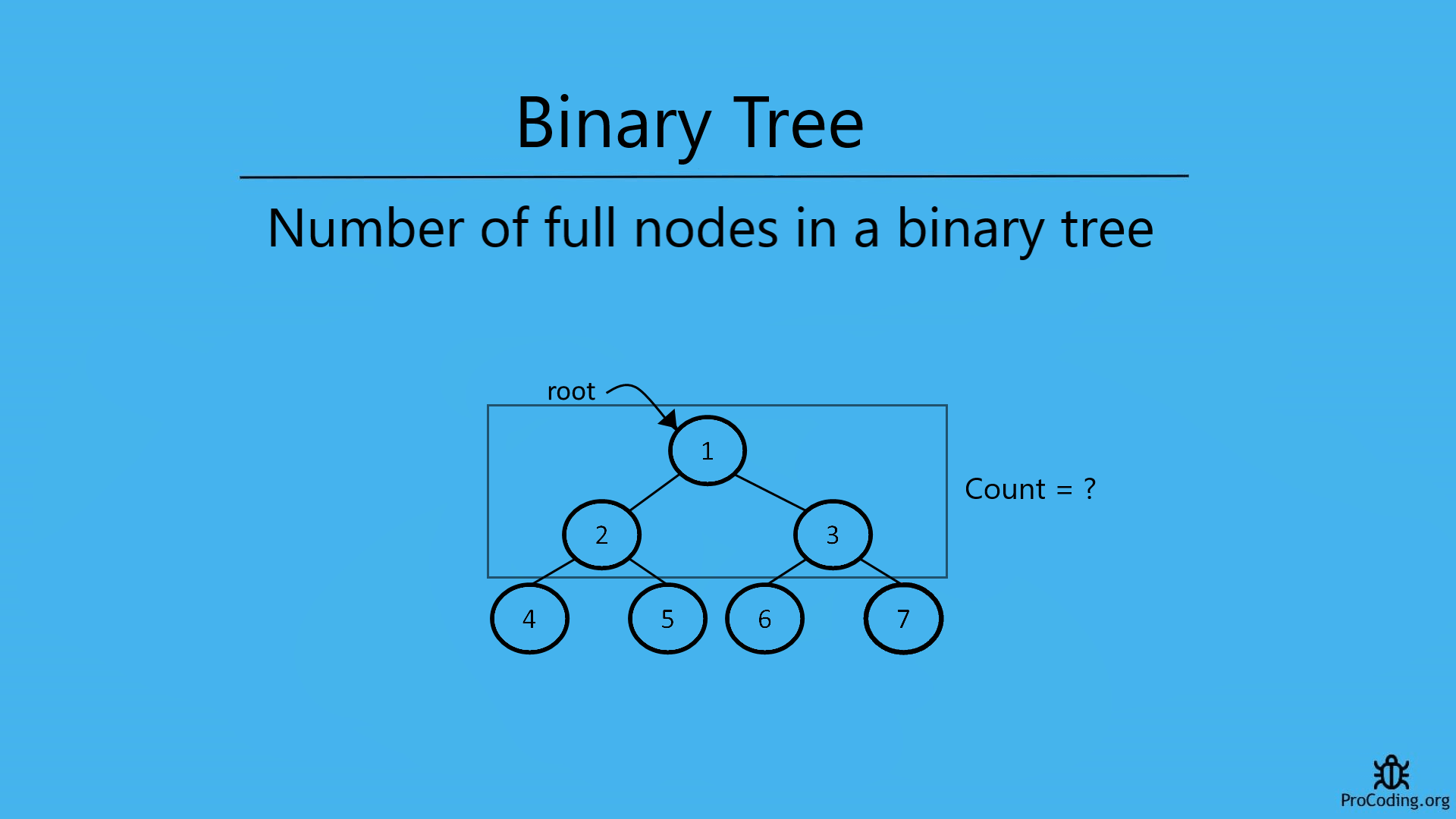# Number of full nodes in a binary tree

Abhishek Kumar Category: Data StructuresTags: datastructures tree binary-tree

Those nodes in the tree which have both the children are known as full nodes i.e., A node is a full node if both left and right child nodes of it are present or not null.

To find the number of full nodes in a binary tree, we have to traverse each node and in the tree and check if the current node is a full node or not and count them one by one.To find the number of full nodes in a binary tree, we have to traverse each node and in the tree and check if the current node is a full node or not and count them one by one.

### Full nodes in a binary tree

Those nodes in the tree which have both children are known as full nodes.

A node is a full node if both left and right child nodes of it are present or not `null`.So, to count all the leaf nodes we have to traverse each node in the tree and count all those nodes which are having both the children i.e, both the children are not `null`.

##### Example -
```  Input:
1
/   \
2     3
/ \   / \
4   5 6   7

Output: 3
```

We can solve this problem in two ways-

### Recursive solution

For recursive solution we have to check each node one by one recursively as we have done in binary tree recursive traversal and count the nodes which are having both the children.

###### Algorithm
Full_Node_Count(root)
``````1. if root is null then
2.    return 0
3. count = 0
4. if root->left is not null and root->right is not null then
5.   count = count + 1
6. left_count = Full_Node_Count(root->left)
7. right_count = Full_Node_Count(root->right)
8. count = count + left_count + right_count
9. return count``````
``````class Node:
def __init__(self, data):
self.left = None
self.data = data
self.right = None

def full_node_count(root):
if root is None:
return 0
count = 0
if root.left and root.right:
count = count + 1
left_count = full_node_count(root.left)
right_count = full_node_count(root.right)
count = count + left_count + right_count
return count

root = Node(1)
root.left = Node(2)
root.right = Node(3)
root.left.left = Node(4)
root.left.right = Node(5)
root.right.left = Node(6)
root.right.right = Node(7)
print(full_node_count(root))``````
``````class Node {
constructor(data) {
this.left = null;
this.data = data;
this.right = null;
}
}

function fullNodeCount(root) {
if (!root) return 0;

let count = 0;
if (root.left && root.right) count++;
const leftCount = fullNodeCount(root.left);
const rightCount = fullNodeCount(root.right);
count = count + leftCount + rightCount;
return count;
}

const root = new Node(1);
root.left = new Node(2);
root.right = new Node(3);
root.left.left = new Node(4);
root.left.right = new Node(5);
root.right.left = new Node(6);
root.right.right = new Node(7);
console.log(fullNodeCount(root));``````

Output

`3`

### Iterative solution

For iterative solution, we have to traverse each node in level order and count the nodes which are not having any child.

###### Algorithm
Full_Node_Count(root)
``````1.  if root is null then return 0
2.  queue.enqueue(root)
3.  count = 0
4.  while queue is not empty
5.      node = queue.dequeue()
6.      if node->left is not null and node->right is not null then
7.          count = count + 1
8.      if node->left is not null then
9.         queue.enqueue(node->left)
10.     if node.right is not null then
11.        queue.enqueue(node->right)
12. return count``````
``````import queue

class Node:
def __init__(self, data):
self.left = None
self.data = data
self.right = None

def full_node_count(root):
if root is None:
return 0

q = queue.Queue()
q.put(root)
count = 0
while not q.empty():
node = q.get()
if node.left and node.right:
count = count + 1
if node.left:
q.put(node.left)
if node.right:
q.put(node.right)
return count

root = Node(1)
root.left = Node(2)
root.right = Node(3)
root.left.left = Node(4)
root.left.right = Node(5)
root.right.left = Node(6)
root.right.right = Node(7)
print(full_node_count(root))``````
``````class Node {
constructor(data) {
this.left = null;
this.data = data;
this.right = null;
}
}

function fullNodeCount(root) {
if (!root) return 0;

const q = [];
q.push(root);
let count = 0;
while (q.length > 0) {
const node = q.shift();
if (node.left && node.right) count++;
if (node.left) q.push(node.left);
if (node.right) q.push(node.right);
}
return count;
}

const root = new Node(1);
root.left = new Node(2);
root.right = new Node(3);
root.left.left = new Node(4);
root.left.right = new Node(5);
root.right.left = new Node(6);
root.right.right = new Node(7);
console.log(fullNodeCount(root));``````

#### Output

Output

`3`

Time complexity: The time complexity of both of these solution will be O(n) because we are just traversing each element of node one by one.

AUTHOR### Abhishek Kumar

Software engineer | Blogger | Keen learner
Python | Django | JavaScript | React | Next.js | Express.js | C
Passionate about learning and experimenting with new things and open to more opportunities and collaborations.